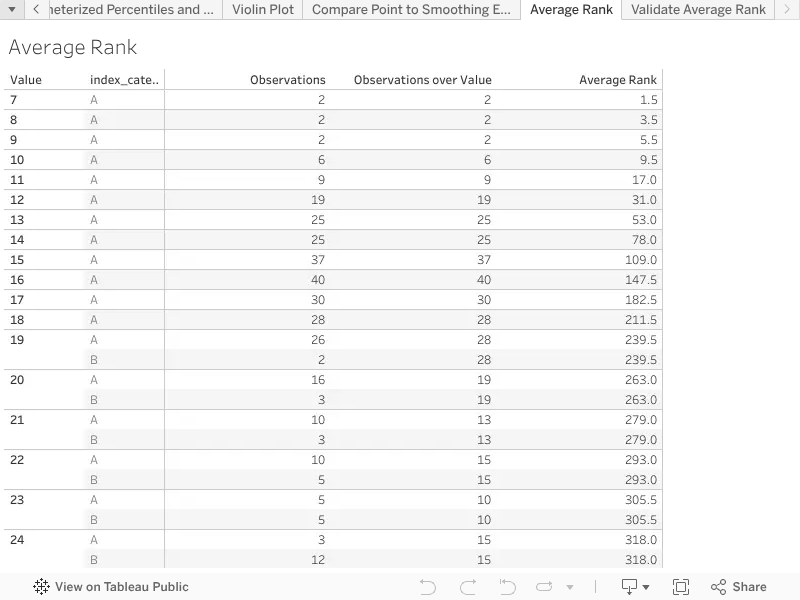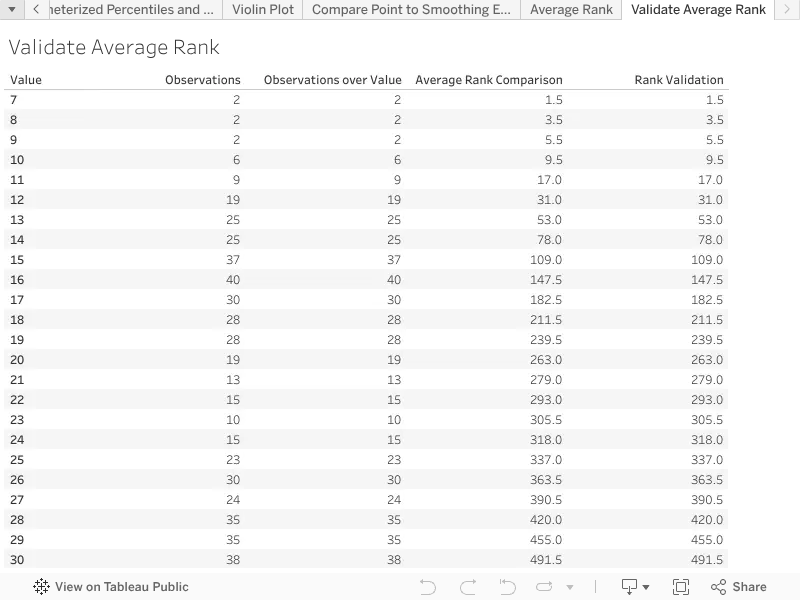# Average Rank from Count Data

Version 20

Description:

All rank tests, such as the Mann-Whitney and the Kruskal-Wallis, assign the average rank to ties, to ensure consistency when summing over consecutive tied ranks, specifically so that the sum of the average rank over a set of ties equals the sum of the ranks as if the ties could be ranked uniquely. In the case of tabulated data the record count SUM([Number of Records]) in a window implicitly measures the number of ties. From simple linear arithmetic progressions we know that the average rank of a set of ties is the simple average of the lowest and highest possible rank, as if all the ties could be sorted uniquely. In the case of tabulated data, the average rank can be expressed in terms of the running sum of the count of ties.

When the windows of equal rank have only one row we can immediately identify the first additive term in the right hand side of the last line as the RUNNING_SUM() table function, and the second additive term as the WINDOW_SUM() table function, constrained to the current window of the addressed ordering. However, in rank tests the windows of equal ranks will always contain more than one row, because we are testing for stochastic dominance of one value in a categorical dimension [CATEGORY] over the other values, according to a ranking specified by an ordering in another field [ORDER]. In this case we have to arbitrage a level of detail calculation, to count the ties, against a table calculation to find the average rank from the tie count, because table calculations cannot be re-addressed or sub-addressed.

Example Calculation:

For the simple case of one row in each window the average rank is the average of the lowest possible rank and the highest possible rank over the ties in the window.

// Assign the average rank using the upper rank of the window,

// and the number of records in the window.

[AVERAGE RANK] := RUNNING_SUM(SUM([Number of Records])) -

(WINDOW_SUM(SUM([Number of Records]), 0, 0) - 1) / 2

For general rank tests with multiple rows in the window of a single rank we use the same look back test for a change of [ORDER] windows that was used to build mosaic plots.

// The average rank within the window is the number of records in the

// previous windows plus half the number of records in the current window,

// starting from one half.

[AVERAGE RANK] := PREVIOUS_VALUE(1 / 2) +

// Add half the number of records in the first window.

IF INDEX() < 2 THEN

ATTR({ FIXED [ORDER] : SUM([Number of Records]) }) / 2

// If this is the first row of a new window add half the previous records,

// and half the current records.

ELSEIF LOOKUP(ATTR([ORDER]), -1) < ATTR([ORDER]) THEN

(

ATTR({ FIXED [ORDER] : SUM([Number of Records]) }) +

LOOKUP(ATTR({ FIXED [ORDER] : SUM([Number of Records]) }), -1)

) / 2

// Skip mid-window rows.

ELSE

0

END

Inputs and Setup:

The average rank requires at least one field that determines the ranking of the windows, in the example the field is called [ORDER].

For the average rank to be non-trivial the table calculation must address the same field that determines the order of ranking, in the example the field is called [ORDER]. The trick to have the average rank calculate properly regardless of the ordering of the pills is to edit the table calculation and choose specific dimensions. Choose all the dimensions but the drag the field the determines the ordering to the top of the list so that it is addressed first. If the average rank within a partition is required, as opposed to over the whole table, additional partitioning fields can be added to the table calculation, provided those fields are also included in the level of detail partition definitions.

The simple table function for the case of one row per window can be used as an effective cross validation of the more complex multi-row level of detail calculation. Empty cells may need to be treated with care.

Related Functions:

SUM(), RUNNING_SUM(), WINDOW_SUM(), PREVIOUS_VALUE(), INDEX(), LOOKUP(), ATTR()

The following table illustrates the average rank calculated from record counts.The next table illustrates the use of the simpler table calculation to cross validate the average rank calculation.The source code to generate the sample data is available in a Jupyter notebook hosted on GitHub.

By passing tabulated average ranks and tie counts to R we can reduce the complexity of the calculation of the rank statistics in the R scripts. This is important because R's rank tests expect granular individual observations (e.g. Wilcoxon, and Kruskal-Wallis), while Tableau only interoperates with R by passing tabulated data through table functions. Thus the calculation of the rank statistic has to be specified in the R script, followed by a call to the appropriate distribution. By having Tableau tabulate the average ranks you will reduce the chance of coding errors in your R scripts, increase the clarity of your R scripts, and depending on your version of Tableau, take advantage of the substantial performance improvements for the mundane tasks, like in memory heap management, available through the Hyper data engine. Rank tests are part of a large family of non-parametric statistics.